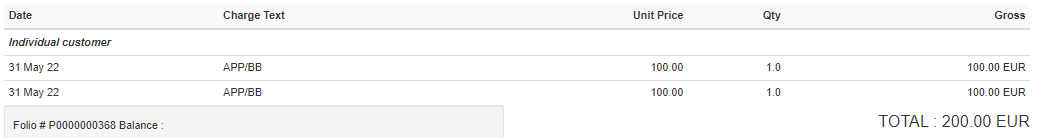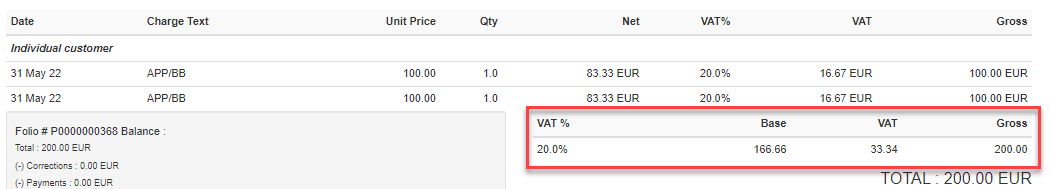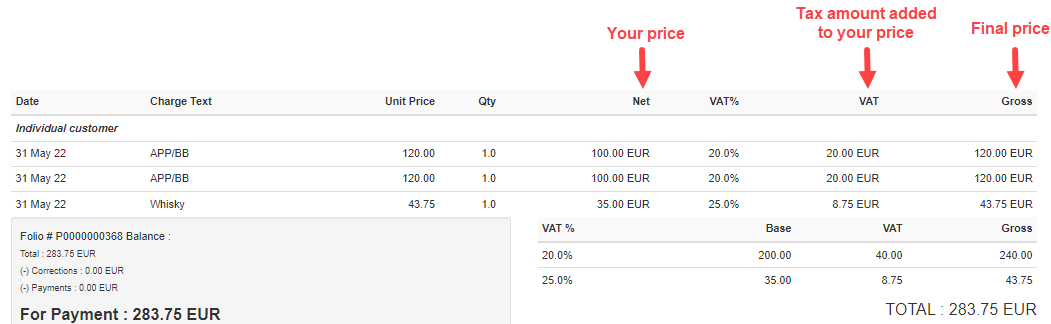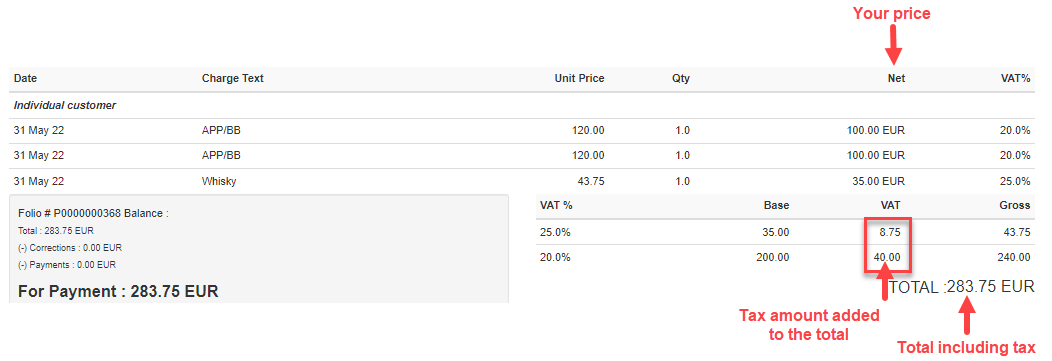# Tax Modes

Modified on: Thu, 10 Nov, 2022 at 2:14 PM

Print

The selection of a tax mode is one of the most important tax settings. The tax type determines how the information will be arranged in your invoices and how the tax will be calculated.

Clock PMS+ provides its users with a wide range of tax modes:

• No tax
• Tax included in prices, rounding - per line
• Tax included in prices, rounding - total
• Tax not included in prices, rounding - per line
• Tax not included in prices, rounding – total
Please note that the different rounding options may result in differences in the invoice total. And the values of the tax rates in Clock PMS+ can have up to 4 decimal places.

## No tax

Under the "No tax" mode the gross and net amounts are the same, coinciding with the rate prices, as no tax is added to these prices.## Tax included in prices, rounding - per line

In the system,  the gross prices are configured. The tax is determined by the prices set in the rates.

In the folio, the tax is calculated the following way:

1. For each folio line, the TAX BASE is calculated as per the following formula: Total/( 1 + Tax%/100). The resulting amount is rounded to the smallest monetary unit (usually 0.01).

Example for 20% Tax and final price of 100 EUR: 100/1.2 = 83.33333…, the rounded amount is equal to 83.33.

2. For each folio line, the TAX is calculated as PRICETAX BASE = TAX.

Example for 20% Tax: 100 - 83.33 = 16.67.

3. In the folio total section, the respective amounts are calculated by summing the lines.

Example for 20% Tax: Two lines, each containing a final price of 100 EUR:

TOTAL TAX BASE = 83.33 + 83.33 = 166.66.

TOTAL TAX = 16.67 + 16.67 = 33.34Note: Tax mode specifics: If you calculate the tax on the basis of the TOTAL amounts, you will not have the same result. Example for 20% Tax: 200/1.2 = 166.66666…, the rounded amount is equal to 166.67. And the resulting tax base amount as per this tax mode is 166.66. See also the other tax mode: 'Tax included in prices, rounding - total'

## Tax included in prices, rounding - total

In the system,  the gross prices are configured. The tax is determined by the prices set in the rates. The difference with the previous mode is the way the tax is charged.

In the folio, the tax is calculated the following way:

1. The folio TOTAL is calculated by summing the gross prices of each line.

Example: Two lines, each containing a gross price of 100 EUR, and their total is: 100 + 100 = 200 EUR

2. On the basis of this TOTAL, the TAX BASE is calculated using the following formula: Total/( 1 + Tax%/100). The resulting amount is rounded to the smallest monetary unit (usually 0.01).

Example for 20% Tax: 200/1.2 = 166.66666…, the rounded amount is 166.67

3. The TAX amount is calculated as TOTALTAX BASE = TAX AMOUNT.

Example for 20% Tax: 200 - 166.67 = 33.33. Thus calculated, the TAX BASE and TAX are presented in the folio total section.

4. Additionally, the tax bases of each line are also calculated, as these amounts are rounded in each line.

Example for 20% Tax: the tax base for each of two lines, containing a final price of 100 EUR, is calculated the following way: Tax base = 100 / 1.2 = 83.3333… = 83.33Note: Tax mode specifics: The sum of the individual tax base amounts of the folio lines DOES NOT correspond to the folio tax base. Example for 20% Tax: The tax base of the whole folio, calculated as per the above formula: Total/( 1 + Tax%/100), is 166.67, and the sum of the tax bases of the individual folio lines is 83.33 + 83.33 = 166.66. See also the other tax mode: 'Tax included in prices, rounding - per line'

## Tax not included in prices, rounding - per line

In the system, the net prices are set. When charging the services, the tax amount is automatically levied on the net prices.

In the folio, the tax is calculated the following way:

1. The tax amount of each line (charge) is calculated on the basis of the following formula:

Tax Value = Bankers' Rounding (Net Value * (Tax Rate / 100))

2. The gross value for each line (charge) is equal to the sum of the net amount and the tax amount in this line.

3. The gross amount of the invoice (invoice total) is equal to the sum of the values in the lines of the Gross amount column.

4. The total tax amount of the invoice is equal to the sum of the values in the lines of the tax column. For each tax rate, the sum of the corresponding tax amounts is shown in the invoice.## Tax not included in prices, rounding – total

In the system, the net prices are set. The tax is charged the following way:

1. No gross value for each line is calculated.

2. The total net amount of the invoice is the sum of the net amounts in each line of the Net column.

3. The total tax amount of the invoice is calculated on the basis of the following formula:

Rounding (Total Net Value * (Tax Rate / 100)). For each tax rate, the sum of the corresponding tax amounts is shown in the invoice.

4. The total gross amount is calculated as per the following formula: Total Gross Amount = Total Net Amount + Total Tax Amount.Note: The round-half-to-even rounding method (Bankers' Rounding) is used for all tax modes.

Did you find it helpful? Yes No

Send feedback